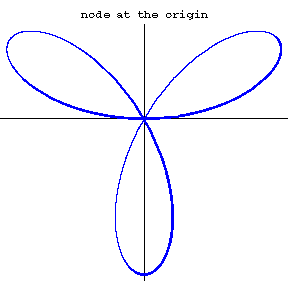# Node

The term which may refer to one of the following:

• A singular (non-smooth) point of an algebraic curve which is a transversal intersection of smooth branches;
• A vertex of the boundary of a nodal domain in the Harmonic analysis (related to the above and also to the [of the node in Physics]);
• A singular point of vector field of a special type (with all eigenvalues of the linear part being to one side of the imaginary axis);
• A point at which the function is evaluated for purposes of interpolation or numeric integration.

## Node of a curve

2010 Mathematics Subject Classification: Primary: 14H20 [MSN][ZBL]

For an algebraic (or analytic) planar curve, the node (or nodal point, also nodal singularity) is a point of self-intersection of smooth branches of the curve.

Example. For the curve $r =s\sin3\varphi$ (in the polar coordinates) the origin of coordinates is a node.Warning. Often it is additionally required that the curve has only transversal self-intersections. In this case at most two smooth branches of the curve can cross each other at any given point, thus the above example will be not a node in this restricted sense.

How to Cite This Entry:
Node. Encyclopedia of Mathematics. URL: http://encyclopediaofmath.org/index.php?title=Node&oldid=30969
This article was adapted from an original article by A.F. Andreev (originator), which appeared in Encyclopedia of Mathematics - ISBN 1402006098. See original article